Search Terms#Technical Trivia by Dr. FB

## Does a wire dipole antenna need a balun?Dr. FB

It is generally well known to insert a balun at the feed point between the coaxial cable and a wire dipole antenna. In the explanations and articles on baluns and theory of balanced/unbalanced, when an unbalanced coaxial cable is connected to a balanced antenna, the current distribution on the left and right antenna element becomes uneven, as shown in Figure 1(b).

It is said that the balance of radiated power will be lost. It is also explained that the figure-8 H-plane radiation pattern of the dipole is distorted due to it, and more radio waves are radiated from the coaxial cable. This seems to cause TVI and BCI, which is a matter of concern to amateur radio operators. Let us examine the explanation with and without a balun through a simple experiment.Figure 1. RF Current distribution on each element

### 1. A wire dipole antenna as I remember

The line that sends RF power from the antenna connector of a radio through the coaxial cable is an unbalanced circuit, and a dipole antenna with 1/4 λ elements stretched to the left and right is a balanced circuit.

Baluns are devices that convert between unbalanced circuits and balanced circuits. Balun is spelled “Balun” in English. It is a word synthesized from parts of the phrase balanced-to-unbalanced.

The balun’s theory may have existed for a long time, but when Dr. FB started amateur radio, the antenna to be built was as shown in Figure 2, and I thought it was a dipole antenna. I did not know the existence of a balun at that time. I enjoyed communicating using this dipole antenna, but I found out that TVI happened in a neighborhood house. At that time, the TVI was greatly eliminated by connecting to the antenna through a balun.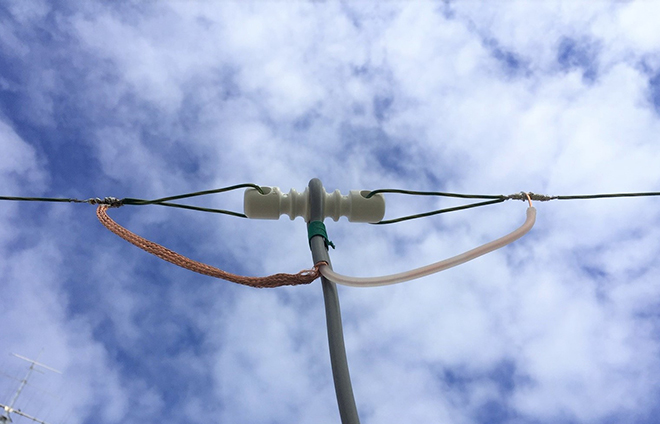Figure 2. Dipole antenna without a balun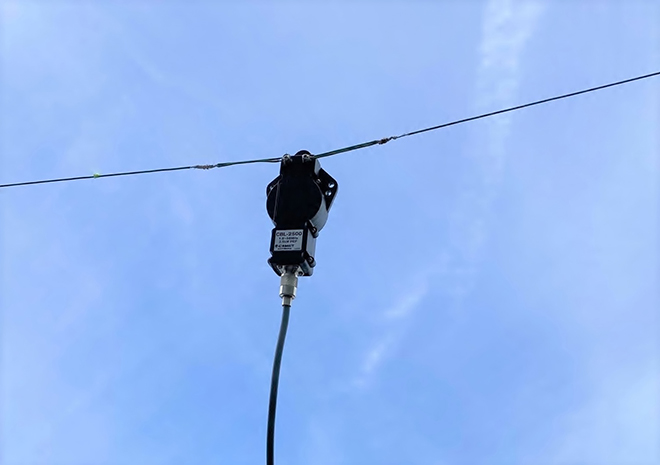Figure 3. Dipole antenna using a balun

### 2. Variety of balun

Figure 4 shows various baluns that I collected for this article. For reference, a balun (b) is made by Bencher, which is famous for the Bencher CW paddle. I thought the Bencher was the maker of CW paddles, but I knew for the first time that Bencher was also making baluns. You can get a lot of information by searching for “Balun” online. From that information, the inside circuit structure of the balun can be roughly known.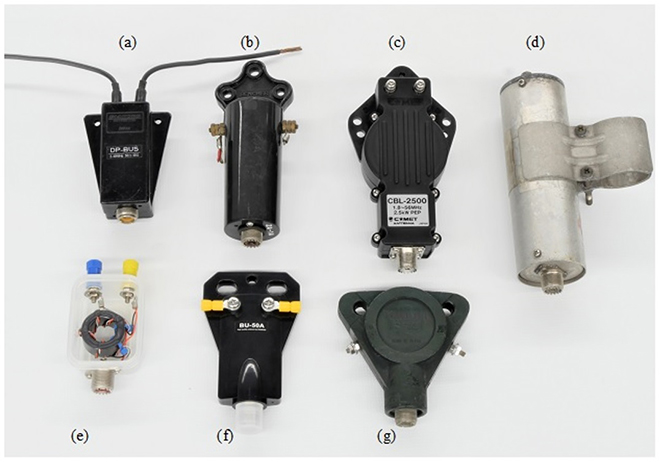Figure 4. Various baluns

### 3. Construction of the balun

Of the baluns shown in Figure 4, I disassembled the balun (a) and examined the insides. Three coils with the same number of turns are tightly wound on the same ferrite bar (core). The circuit is shown in Figure 5. The RF power generated by the radio is supplied to balun coils A and B through the coaxial cable. RF power is also induced in coil C by the electromagnetic induction action, so the RF power induced in coils B and C is supplied to the antenna. In other words, you can see that the power generated by the radio is directly supplied to the dipole antenna through the balun.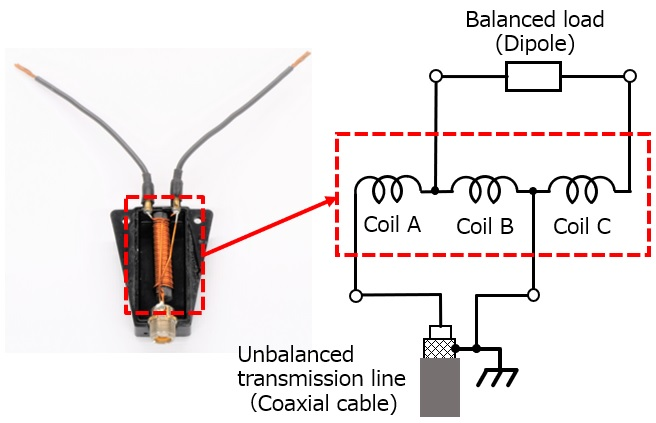Figure 5. Schematic of the balun and its circuitry

### 4. Consideration of a wire dipole without a balun

A balanced line is a signal transmission line consisting of two conductors of the same type, the same impedances along their lengths and thickness, and the same impedances to other circuits. In a coaxial cable, the core wire (center conductor) is protected from external noise by the surrounding braid (shield wire). The braided wire is different from the core wire, so the amount of noise is obviously worse than on the core wire, and the physical shape is also different from the core wire. The characteristics of the two wires that transmit power, the coaxial cable, are not the same. That is, the coaxial cable that we usually use, is an unbalanced line.

In the dipole with a balun shown in Figure 1(a), from the coaxial cable to the balun, the RF power is supplied by the core wire and the braided wire, but after passing through the balun, it becomes symmetrical as shown in Figure 5. RF power is output from both ends of coil B and coil C, and the power is radiated through the elements of the dipole.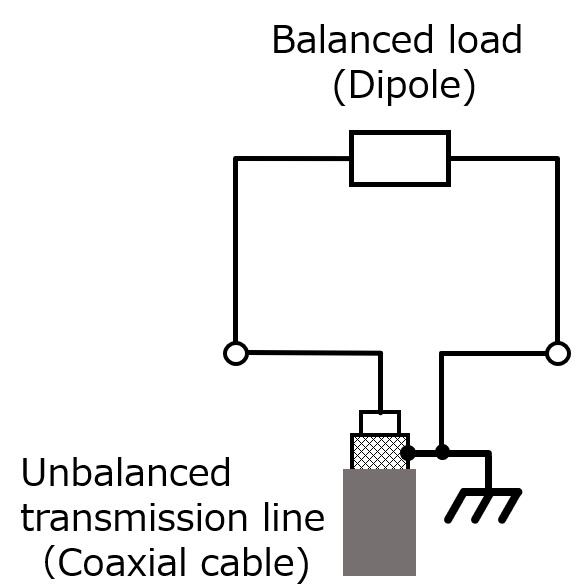Figure 6. Schematic of dipole antenna without a balun

### 5. Preparations of experiment

I made two types of dipole antenna with and without a balun this time, and the RF current flowing through the elements of the dipole is measured with a RF ammeter to create a current distribution diagram. For the transmitted RF signals, the output of an antenna analyzer with very low power was used. The experiment was conducted in the 28 MHz band.

The element length of the dipole antenna is 1/4 λ on one side and 1/4 λ on the other side, and the total of the left and right element lengths is 1/2 λ.Let us calculate the element length. Here, v=3 x 108 (m) as the verocity of wave and f=operating frequency (MHz), but for ease of calculation, λ=300/28.5. The wavelength is 10.5 (m) as shown below.The length of each element of the dipole antenna is 1/4 λ of the wavelength, so it is 2.6 m for a 28.5 MHz dipole.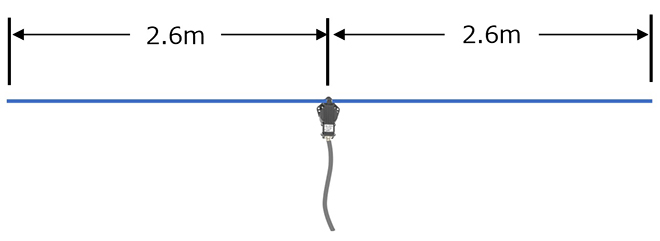Figure 7. Calculation of element length of half-wave dipole

### 6. The result of the experiment

An RF ammeter was used to measure the magnitude of the current flowing through each element (1) when the balun was used in the dipole antenna, and (2) when the balun was not used. The RF ammeter for measuring is shown in Figure 8. With this measuring instrument, the magnitude of the RF current flowing through the element is converted into a voltage, and the value is displayed on the voltmeter of the digital multimeter. Please understand that the measured value represents the relative value of the RF current flowing through the element, and is not an accurate absolute value.Figure 8. A scene of the RF current is being measured

(1) RF Current distribution under a balun connected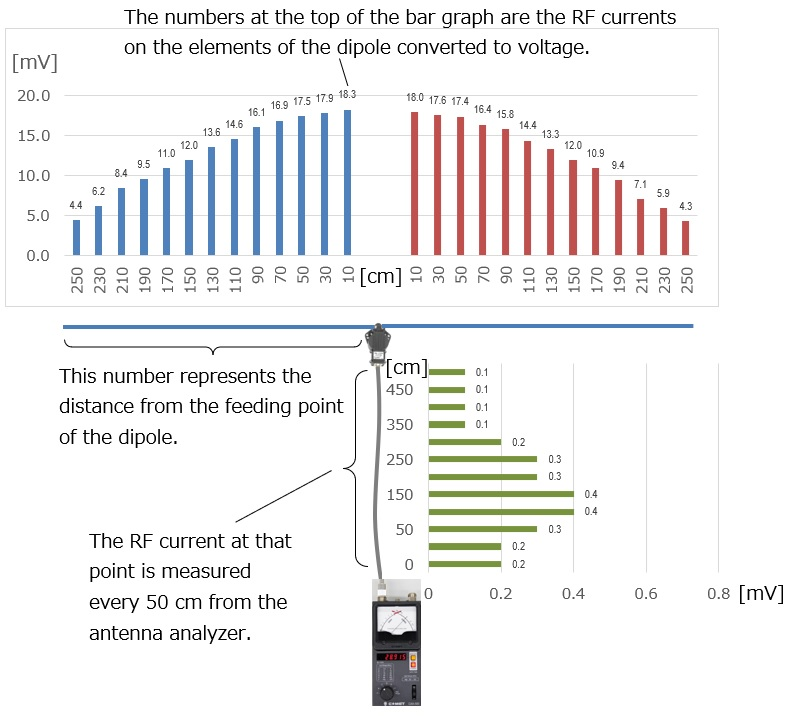Figure 9. Graph of RF current distribution under a balun connected

(2) RF Current distribution under a no balun connectedFigure 10. Graph of RF current distribution under no balun connected

### 7. Consideration of the RF current on antenna element

(1) Consideration of current level flowing in each element of dipole antenna with a balun

Figure 11 is an excerpt from Figure 9. A bar graph shows the magnitude of RF current flowing through the dipole element. The vertical axis shows the measured current level with a voltmeter. As you can see from the figure, the areas enclosed by the dotted lines on the left and right are almost the same. A sum of the measured current levels at each point is 166.4 mV for blue and 162.5 mV for red respectively, and you can see that the numbers are almost equal.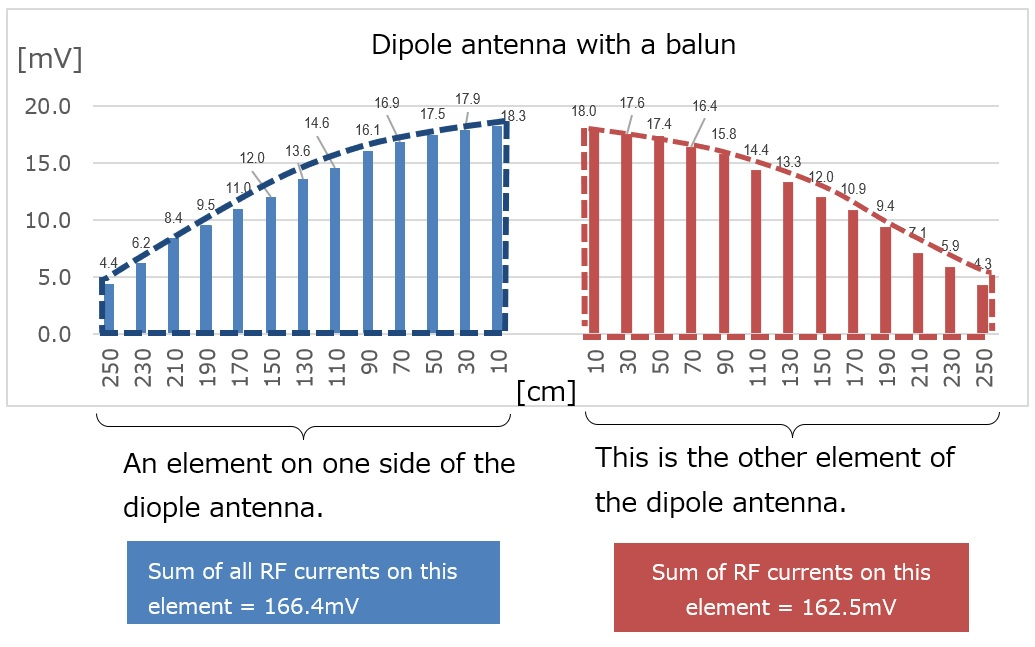Figure 11. RF Current distribution (with a balun)

(2) Consideration of current level flowing in each element of dipole antenna without a balun

Figure 12 is an excerpt from Figure 10. Similar to the above, the bar graph shows the magnitude of the RF current flowing through the dipole element. As you can see from the figure, the area enclosed by the dotted lines on the left and right is large on the red side and small on the blue side. The sum of the current values at each point is 141.1 mV for blue and 166.1 mV for red, and the numbers show that the difference is much larger than the difference when the balun is used.

As I briefly explained in the beginning of this article, I confirmed that a dipole without a balun explains that the electric power radiated from the left and right elements is out of balance in this experiment. However, I have not been able to verify that the figure-8 radiation pattern is distorted. If the energy radiated from the left and right elements is different, you can easily imagine that the directional pattern of the dipole is also affected.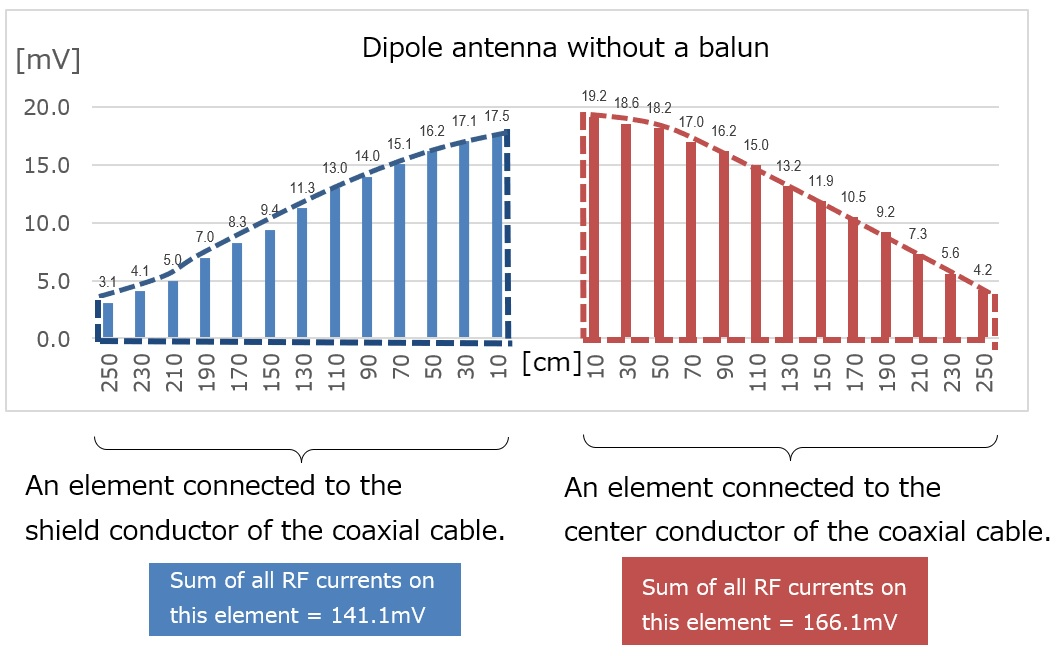Figure 12. RF Current distribution (without a balun)

### 8. Considerations of the RF current on the coaxial cable

Figure 13 shows the current level flowing in the coaxial cable. It is an excerpt from Figures 9 and 10. If you look at the two graphs, you can see that there is a clear difference. The level of the graph on the left with the balun connected is smaller than that on the right. In other words, when the coaxial cable and the elements are directly connected, RF current seems to flow in the coaxial cable. Originally, the coaxial cable serves as a transmission path for radio waves, but as you can see from the results of this experiment, the RF frequencies are also radiated from the coaxial cable.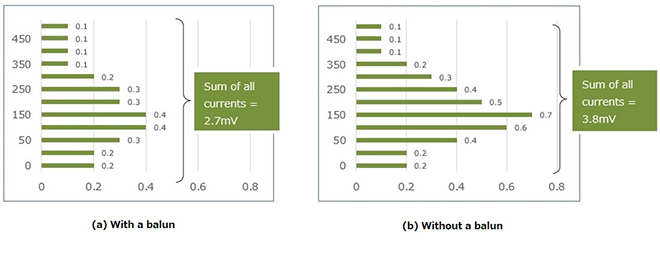Figure 13. RF current distributions on the coaxial cable

### 9. Summary

I found that there is a difference in the RF energy radiated from the left and right elements of the dipole antenna without a balun. Speculation in various books is that this difference is the cause of the disturbance of the directional radiated pattern.

Radiation of RF energy from the coaxial cable was confirmed both when a balun was used and when it was not used. However, the graph in Figure 13 shows that when a balun is not used, about 1.4 times more energy is radiated than when a balun was used.

Originally, the RF energy that should be radiated from the antenna but it is also radiated from the coaxial cable, which could have caused TVI or BCI. My experience is proof of this phenomenon. When I inserted the balun that I got from an OM, the TVI of the neighborhood was suppressed.

Finally, the electric field strength at the point away from the antenna was measured. Due to the installation of the antenna used in the experiment and the environment at the measurement point, the electric field strength of the measurement was erratic and unstable, and the experiments did not proceed as they did in VHF or UHF. As a result, I could not get the data, and I do not know which antenna with a balun or without a balun would be better.

FBDX